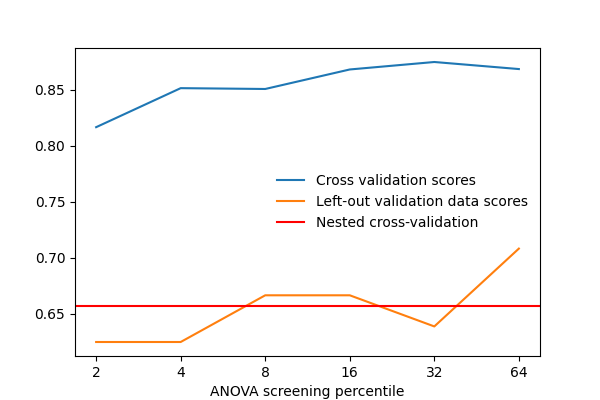# 9.3.10. Setting a parameter by cross-validation¶

Here we set the number of features selected in an Anova-SVC approach to maximize the cross-validation score.

After separating 2 sessions for validation, we vary that parameter and measure the cross-validation score. We also measure the prediction score on the left-out validation data. As we can see, the two scores vary by a significant amount: this is due to sampling noise in cross validation, and choosing the parameter k to maximize the cross-validation score, might not maximize the score on left-out data.

Thus using data to maximize a cross-validation score computed on that same data is likely to optimistic and lead to an overfit.

The proper approach is known as a “nested cross-validation”. It consists in doing cross-validation loops to set the model parameters inside the cross-validation loop used to judge the prediction performance: the parameters are set separately on each fold, never using the data used to measure performance.

For decoding task, in nilearn, this can be done using the `nilearn.decoding.Decoder` object, that will automatically select the best parameters of an estimator from a grid of parameter values.

One difficulty is that the Decoder object is a composite estimator: a pipeline of feature selection followed by Support Vector Machine. Tuning the SVM’s parameters is already done automatically inside the Decoder, but performing cross-validation for the feature selection must be done manually.

## 9.3.10.1. Load the Haxby dataset¶

```from nilearn import datasets
# by default 2nd subject data will be fetched on which we run our analysis
haxby_dataset = datasets.fetch_haxby()
fmri_img = haxby_dataset.func

# print basic information on the dataset
print('Functional nifti image (4D) are located at: %s' % haxby_dataset.func)

import pandas as pd
y = labels['labels']

# Keep only data corresponding to shoes or bottles
from nilearn.image import index_img

```

Out:

```Mask nifti image (3D) is located at: /home/nicolas/nilearn_data/haxby2001/mask.nii.gz
Functional nifti image (4D) are located at: /home/nicolas/nilearn_data/haxby2001/subj2/bold.nii.gz
```

## 9.3.10.2. ANOVA pipeline with `nilearn.decoding.Decoder` object¶

Nilearn Decoder object aims to provide smooth user experience by acting as a pipeline of several tasks: preprocessing with NiftiMasker, reducing dimension by selecting only relevant features with ANOVA – a classical univariate feature selection based on F-test, and then decoding with different types of estimators (in this example is Support Vector Machine with a linear kernel) on nested cross-validation.

```from nilearn.decoding import Decoder
# Here screening_percentile is set to 2 percent, meaning around 800
# features will be selected with ANOVA.
smoothing_fwhm=4, standardize=True,
screening_percentile=2)
```

## 9.3.10.3. Fit the Decoder and predict the responses¶

As a complete pipeline by itself, decoder will perform cross-validation for the estimator, in this case Support Vector Machine. We can output the best parameters selected for each cross-validation fold. See https://scikit-learn.org/stable/modules/cross_validation.html for an excellent explanation of how cross-validation works.

First we fit the Decoder

```decoder.fit(fmri_niimgs, y)
for i, (param, cv_score) in enumerate(zip(decoder.cv_params_['shoe']['C'],
decoder.cv_scores_['shoe'])):

print("Fold %d | Best SVM parameter: %.1f with score: %.3f" % (i + 1,
param, cv_score))
# Output the prediction with Decoder
y_pred = decoder.predict(fmri_niimgs)
```

Out:

```Fold 1 | Best SVM parameter: 100.0 with score: 0.901
Fold 2 | Best SVM parameter: 100.0 with score: 0.918
Fold 3 | Best SVM parameter: 1.0 with score: 0.738
Fold 4 | Best SVM parameter: 100.0 with score: 0.749
Fold 5 | Best SVM parameter: 100.0 with score: 0.736
```

## 9.3.10.4. Compute prediction scores with different values of screening percentile¶

```import numpy as np
screening_percentile_range = [2, 4, 8, 16, 32, 64]
cv_scores = []
val_scores = []

for sp in screening_percentile_range:
smoothing_fwhm=4, cv=3, standardize=True,
screening_percentile=sp)
decoder.fit(index_img(fmri_niimgs, session < 10),
y[session < 10])
cv_scores.append(np.mean(decoder.cv_scores_['bottle']))
print("Sreening Percentile: %.3f" % sp)
print("Mean CV score: %.4f" % cv_scores[-1])

y_pred = decoder.predict(index_img(fmri_niimgs, session == 10))
val_scores.append(np.mean(y_pred == y[session == 10]))
print("Validation score: %.4f" % val_scores[-1])
```

Out:

```Sreening Percentile: 2.000
Mean CV score: 0.8107
Validation score: 0.6111
Sreening Percentile: 4.000
Mean CV score: 0.8493
Validation score: 0.3889
Sreening Percentile: 8.000
Mean CV score: 0.8437
Validation score: 0.4444
Sreening Percentile: 16.000
Mean CV score: 0.8389
Validation score: 0.5000
Sreening Percentile: 32.000
Mean CV score: 0.8059
Validation score: 0.4444
Sreening Percentile: 64.000
Mean CV score: 0.8141
Validation score: 0.4444
```

## 9.3.10.5. Nested cross-validation¶

We are going to tune the parameter ‘screening_percentile’ in the pipeline.

```from sklearn.model_selection import KFold
cv = KFold(n_splits=3)
nested_cv_scores = []

for train, test in cv.split(session):
y_train = np.array(y)[train]
y_test = np.array(y)[test]
val_scores = []

for sp in screening_percentile_range:
smoothing_fwhm=4, cv=3, standardize=True,
screening_percentile=sp)
decoder.fit(index_img(fmri_niimgs, train), y_train)
y_pred = decoder.predict(index_img(fmri_niimgs, test))
val_scores.append(np.mean(y_pred == y_test))

nested_cv_scores.append(np.max(val_scores))

print("Nested CV score: %.4f" % np.mean(nested_cv_scores))
```

Out:

```Nested CV score: 0.5880
```

## 9.3.10.6. Plot the prediction scores using matplotlib¶

```from matplotlib import pyplot as plt
from nilearn.plotting import show

plt.figure(figsize=(6, 4))
plt.plot(cv_scores, label='Cross validation scores')
plt.plot(val_scores, label='Left-out validation data scores')
plt.xticks(np.arange(len(screening_percentile_range)),
screening_percentile_range)
plt.axis('tight')
plt.xlabel('ANOVA screening percentile')

plt.axhline(np.mean(nested_cv_scores),
label='Nested cross-validation',
color='r')

plt.legend(loc='best', frameon=False)
show()
```Total running time of the script: ( 1 minutes 33.025 seconds)

Estimated memory usage: 915 MB

Gallery generated by Sphinx-Gallery